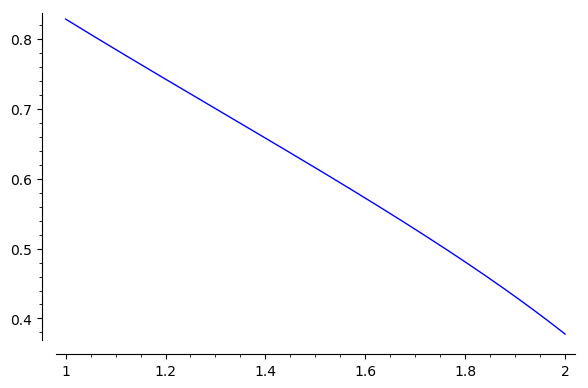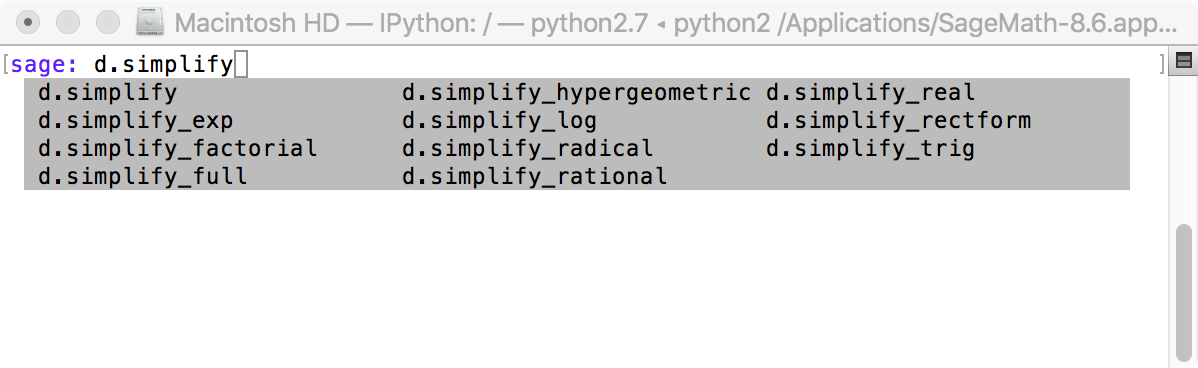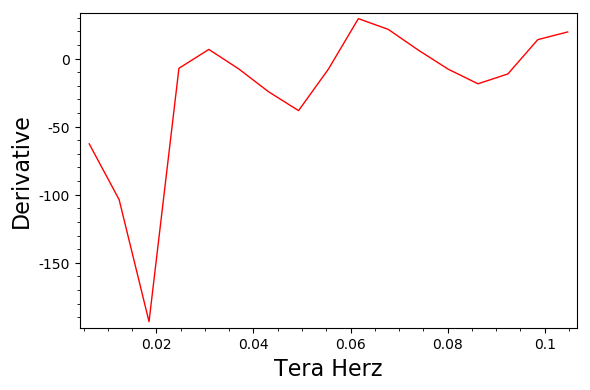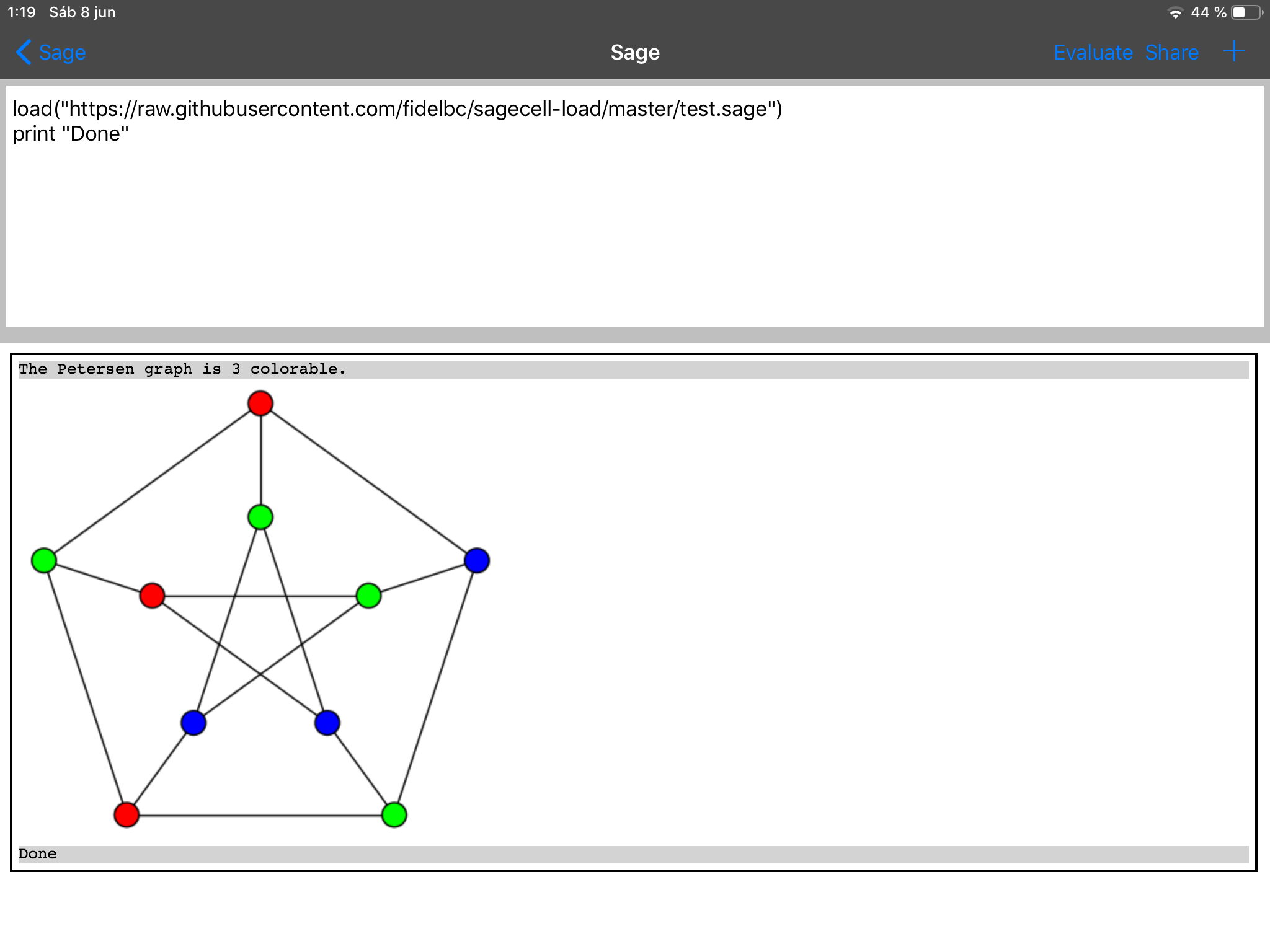2019-07-24 04:41:53 -0500 commented question How to solve Sturm-Liouville problems in SageMath? I think that anything which can be computed in Julia, Octave or Scilab can also be computed in SageMath. So I would suggest to post the code you use to solve the problem in one of these languages, e.g. in Octave. In this way, you could get help to translate it to SageMath. 2019-07-21 09:35:46 -0500 received badge ● Nice Answer (source) 2019-07-18 16:37:00 -0500 answered a question Latex text in notebook and return lines In the notebook, you can display text using HTML instead of LaTeX: text = """ Late at night, guards on the battlements of Denmark's Elsinore castle
and the guards and Horatio decide to tell Hamlet. """ show(html(text))  This allows a better formatting using HTML and CSS. Note, for example, in the above text, that each instance of "Hamlet" is displayed in boldface. 2019-07-04 20:12:45 -0500 received badge ● Nice Answer (source) 2019-07-02 06:35:58 -0500 received badge ● Nice Answer (source) 2019-07-02 04:47:12 -0500 answered a question Octave-like plot function, or, how to plot sequence of points? You can use the list_plot function: x = [1, 5, 7, 8, 8.7, 10, 13, 15] y = [2, 8, 13, 10, 9, 6.3, 2, -1] list_plot(zip(x,y), plotjoined=True)  Se the docs for a complete reference. 2019-07-01 18:36:27 -0500 answered a question Numerical integration and plot failing A different approach that works even in a Python 2 based Sage: sage: var("y,z"); sage: cauchy(z) = y.subs(solve(z*y^3 + y^2 - 2*z*y + 2, y)) sage: F(z) = arctan2(imag(cauchy(z)),real(cauchy(z))) sage: numerical_integral(F, 1, 2) (0.6116545934235456, 6.790730127365591e-15) sage: plot(F(z),(z,1,2))The plot is really slow. It may take more than 20 seconds. See this SageMath cell. 2019-07-01 10:46:59 -0500 answered a question Spanish numbers using LaTeX I will provide a parcial answer to the question. Let us consider the following LaTeX code: \documentclass[12pt]{article} \usepackage[T1]{fontenc} \usepackage[utf8]{inputenc} \usepackage[spanish,es-noshorthands]{babel} \usepackage{amsmath} \usepackage{siunitx} \usepackage{sagetex} \begin{document} \begin{sagesilent} def NumWrap(number): if number in ZZ or number.parent()==QQ: return r"\num{"+ str(number) + "}" else: return r"\num{"+ str(float(number)) + "}" def MatWrap(m): s = r"\begin{pmatrix} " for nrow in range(m.nrows()): for ncol in range(m.ncols()-1): s += NumWrap(m[nrow,ncol]) + " & " s += NumWrap(m[nrow,-1]) if nrow 2: B = (1-t)**3*vector(path)+3*t*(1-t)**2*vector(path)+3*t**2*(1-t)*vector(path[-2])+t**3*p0 G = P3D.parametric_plot3d(list(B), (0, 1), **plot_options) else: G = line3d([path, p0], **line_options) for curve in path[1:]: if len(curve) > 1: p1 = vector(curve) p2 = vector(curve[-2]) p3 = vector(curve[-1]) B = (1-t)**3*p0+3*t*(1-t)**2*p1+3*t**2*(1-t)*p2+t**3*p3 G += P3D.parametric_plot3d(list(B), (0, 1), **plot_options) else: G += line3d([p0,curve], **line_options) p0 = curve[-1] return G  This code defines my_bezier3d, which has exactly the same syntax as bezier3d, but it admits two additional keywords: radius and plot_points. So, we can now use it: path_1 = [[(0,0,0),(1,0,0),(1,1,0)], [(0,1,0),(0,0,1),(1,1,1)], [(0,1,1)]] path_2 = [[(1,0,1),(0.1,0.9,0.7),(0.1,0.9,0.3),(1,0,0)]] path_3 = [[(0,1,0),(0.7,0.9,0.2),(1,0.8,0.4),(0.6,0.4,0.6),(0.5,0,1)], [(0,0,1),(0,0,0.5),(0.5,0.2,0)]] curve_1 = my_bezier3d(path_1) curve_2 = my_bezier3d(path_2, color='green', radius=0.03) curve_3 = my_bezier3d(path_3, color='red', radius=0.01, plot_points=25) show(curve_1+curve_2+curve_3, viewer='threejs')  See the resulting graphic in this SageMath Cell. 2019-06-22 11:07:20 -0500 answered a question Best way to really simplify ugly formulas If you type sage: d.simplify  and the tab key, you will see a pop-up frame showing different ways to complete that command to simplify d:Select one of them, say simplify_full, add ? and press the return key to see the associated help: sage: d.simplify_full? Docstring: Apply "simplify_factorial()", "simplify_rectform()", "simplify_trig()", "simplify_rational()", and then "expand_sum()" to self (in that order). ALIAS: "simplify_full" and "full_simplify" are the same. EXAMPLES: sage: f = sin(x)^2 + cos(x)^2 sage: f.simplify_full() 1 sage: f = sin(x/(x^2 + x)) sage: f.simplify_full() sin(1/(x + 1)) ...........................  In this case, since d contains radicals, you may try another method: sage: d.canonicalize_radical()  which is the actual form of the deprecated simplify_radical. Check if the resulting simplification is good enough for you. 2019-06-20 14:10:09 -0500 commented answer How to use solve() for a system of equations using matrices? Please, see the update. 2019-06-19 12:40:59 -0500 answered a question How to make formal variables with more than one index? Consider the following code: sage: m, n = 3, 2 sage: g = matrix(m+1, n+1, [var("g_{}{}".format(i,j), ....: latex_name="g_{{{}{}}}".format(i,j)) ....: for i in [0..m] for j in [0..n]]) sage: g [g_00 g_01 g_02] [g_10 g_11 g_12] [g_20 g_21 g_22] [g_30 g_31 g_32]  It creates a matrix g of symbolic variables g_00, g_01, etc. You can access each variable either by g_ij or g[i,j]. Edit. To cope with a more general case you can use a dictionary, for example: sage: m, n, p = 3, 2, 2 sage: g = {(i,j,k): var("g_{}{}{}".format(i,j,k), ....: latex_name="g_{{{}{}{}}}".format(i,j,k)) ....: for i in [0..m] for j in [0..n] for k in [0..p]} sage: g {(0, 0, 0): g_000, (0, 0, 1): g_001, (0, 0, 2): g_002, (0, 1, 0): g_010, (0, 1, 1): g_011, ................................. (3, 2, 0): g_320, (3, 2, 1): g_321, (3, 2, 2): g_322}  Variables can be accessed by g_ijk or g[(i,j,k)]. 2019-06-18 12:13:59 -0500 received badge ● Good Answer (source) 2019-06-18 06:38:11 -0500 received badge ● Nice Answer (source) 2019-06-18 05:39:24 -0500 answered a question Testing if the entries of a matrix of rational vectors are actually integers Try this example: sage: set_random_seed(100); # to be able to reproduce this example sage: A = random_matrix(QQ, 5, 4, num_bound=20, den_bound=4); A [ 0 -1 19/3 -1/4] [ -1/2 8 1 -5/2] [ -7/2 -9 -5 3/2] [-11/4 -1/4 -8 -15/4] [ -9 14/3 8 4/3] sage: all(map(lambda x: x.is_integer(),A.list())) False  Hope that helps. Edit. I have found the denominator method, which yields the lowest common denominator of the matrix elements. So, all entries are integers if and only if such denominator is 1. As a continuation of the above example, we have: sage: A.denominator()==1 False  2019-06-13 03:41:18 -0500 received badge ● Good Answer (source) 2019-06-12 09:40:33 -0500 commented answer Derivative of a CSV data set Yes, just add np.savetxt("deriv.csv", zip(data[1:,0],t), delimiter=",")  at the end of the last block of code. See https://docs.scipy.org/doc/numpy/reference/generated/numpy.savetxt.html for details on savetext. 2019-06-12 08:46:34 -0500 received badge ● Nice Answer (source) 2019-06-12 06:06:47 -0500 commented question Derivative of a CSV data set I think that the problem was the lack of a pure Python equivalent of the Matlab diff function, which can be replaced with a list comprehension. Anyway, you are right, things are more easily and efficiently done with Numpy. 2019-06-11 20:42:44 -0500 answered a question Derivative of a CSV data set Please, try the following code: import csv with open("test.csv") as csv_file: data = list(csv.reader(csv_file)) ndata = len(data) data = map(lambda x: [float(x),float(x)], data) data = matrix(data) P = list_plot(data, plotjoined=True, color="blue", frame=True, axes=False, axes_labels=["Tera Herz", "Absorbance"]) P.show() t = [(data[i+1,1]-data[i,1])/(data[i+1,0]-data[i,0]) for i in range(ndata-1)] l = list_plot(zip(data[1:,0].list(),t), plotjoined=True, color='red', frame=True, axes=False, axes_labels=["Tera Herz", "Derivative"]) l.show()  Here, test.csv is a file with the data you provided. As an alternative, with a more clear code but perhaps slower: import csv with open("test.csv") as csv_file: data = csv.reader(csv_file) xx, yy = [], [] for row in data: xx.append(float(row)) yy.append(float(row)) ndata = len(xx) P = list_plot(zip(xx,yy), plotjoined=True, color="blue", frame=True, axes=False, axes_labels=["Tera Herz", "Absorbance"]) P.show() t = [(yy[i+1]-yy[i])/(xx[i+1]-xx[i]) for i in range(ndata-1)] l = list_plot(zip(xx[1:],t), plotjoined=True, color="red", frame=True, axes=False, axes_labels=["Tera Herz", "Derivative"]) l.show()  Edited. Following the advice of @Iguananaut, let us use Numpy. The code becomes: import numpy as np data = np.loadtxt("test.csv", delimiter=",") P = list_plot(data, plotjoined=True, color="blue", frame=True, axes=False, axes_labels=["Tera Herz", "Absorbance"]) P.show() t = np.diff(data[:,1])/np.diff(data[:,0]) l = list_plot(zip(data[1:,0],t), plotjoined=True, color='red', frame=True, axes=False, axes_labels=["Tera Herz", "Derivative"]) l.show()  In any case I get the following pictures:2019-06-08 14:00:05 -0500 received badge ● Good Answer (source) 2019-06-08 13:59:07 -0500 received badge ● Nice Answer (source) 2019-06-08 13:58:23 -0500 received badge ● Nice Answer (source) 2019-06-07 18:36:28 -0500 answered a question iPad app pre-load user definitions? The SageMath app sends the code to a Sagecell server, retrieves the output and presents it in the iPad screen. Hence this app has the same limitations as Sagecell. You can not read a local file (in your computer or iPad), but you can read files accessible in the web via their URL. See https://ask.sagemath.org/question/39295/how-can-sagecell-read-text-file-from-url. See also, for example, https://github.com/fidelbc/sagecell-load and test the loading of the Sage file published there. I get this in my iPad:2019-06-07 15:47:46 -0500 answered a question positive values of polynomials From the examples in the O.P., I assume that we are dealing with homogeneous quadratic polynomials, that is, with quadratic forms. Let us state the problem: given a quadratic form $p$ in $\mathbb{R}^n$, determine the truth value of the proposition (P) there exists $\mathbf{x}\in\mathbb{R}_+^n$ such that $p(\mathbf{x})>0$. Here, $\mathbb{R}_+$ stands for the set of positive real numbers. One can apply, at least, one of the following tests: Test 1. Let $A$ be the symmetric matrix associated to $p$, i.e., $p(\mathbf{x})=\mathbf{x}^TA\mathbf{x}$. Compute the eigenvalues of $A$. If all the eigenvalues are less than or equal to $0$, then (P) is false. If there exists a positive eigenvalue $\lambda$ with an associated eigenvector $\mathbf{v}\in\mathbb{R}_+^n$, then (P) is true. Note that this test does not cover all the possibilities, since it only checks sufficient conditions. Test 2. Compute the maximum of $p$ on the set $D=[0,1]^n$. Then (P) is true if and only if such a maximum is positive. Test 1 can be implemented through the eigenvalues() and eigenvectors_right() methods for matrices. Test 2 can use the minimize_constrained function, already presented in @dan_fulea’s answer. Please note that maximize $p$ is equivalent to minimize $-p$. Let us apply them to the given polynomials. Example 1. Let $p=-x^2 - y^2 - z^2 + xy + xz + yz$. We apply Test 1: sage: var("x,y,z"); sage: A = matrix([[-1,1/2,1/2],[1/2,-1,1/2],[1/2,1/2,-1]]) sage: A.eigenvalues() [0, -3/2, -3/2]  By Test 1, (P) is false, since all the eigenvalues are less than or equal to $0$. We could also apply Test 2: sage: var("x,y,z"); sage: p = -x^2 - y^2 - z^2 + x*y + x*z + y*z sage: sol = minimize_constrained(-p, [[0,1]]*3, [0.1,0.9,0.5]) sage: print "Maximum is", p(*sol), "attained at", sol Maximum is 0.0 attained at (0.4999999998931121, 0.5000000001068878, 0.5)  Since the maximum is not greater than $0$, by Test 2, (P) is false. The second argument of minimize_constrained is a list of the intervals where $x$, $y$ and $z$ should be, that is, $[0,1]$ for each variable. The last argument is a starting point of the iterative minimization process. In this example, if we take a different starting point, the maximum is also $0$, but it can be reached at a different point ($p$ is $0$ on the line $x=y=z$). Example 2. Let $q= -x^2 - y^2 - z^2 + (3/2)(xy + xz + yz)$. We first use Test 1: sage: var("x,y,z"); sage: A = matrix([[-1,3/4,3/4],[3/4,-1,3/4],[3/4,3/4,-1]]) sage: A.eigenvalues() [1/2, -7/4, -7/4] sage: A.eigenvectors_right() [(1/2, [(1, 1, 1)], 1), (-7/4, [(1, 0, -1), (0, 1, -1)], 2)]  The matrix $A$ has one positive eigenvalue, $\lambda=1/2$, with an associated eigenvector $\mathbf{v}=(1,1,1)$ belonging to $\mathbb{R}_+^n$. Hence, (P) is true. Let us now apply Test 2: sage: var("x,y,z"); sage: q = -x^2 - y^2 - z^2 + (3/2)*(x*y + x*z + y*z) sage: sol = minimize_constrained(-q,[[0,1]]*3, [0.1,0.9,0.5]) sage: print "Maximum is", q(*sol), "attained at", sol Maximum is 1.5 attained at (1.0, 1.0, 1.0)  Since the maximum is positive, by Test 2, (P) is true. Rationale for Test 1. If $A$ does not have a positive eigenvalue, then $A$ is semidefinite negative, and so $p(\mathbf{x})=\mathbf{x}^TA\mathbf{x}\leq 0$ for all $\mathbf{x}\in\mathbb{R}^n$. Consequently, (P) is false. Likewise, if there exists a positive eigenvalue $\lambda$ with an associated eigenvector $\mathbf{v}\in\mathbb{R}_+^n$, then $p(\mathbf{v})=\mathbf{v}^TA\mathbf{v}=\lambda\mathbf{v}^T\mathbf{v}=\lambda\lVert\mathbf{v}\rVert^2>0$. Hence (P) is true. Rationale for Test 2. Since $p$ is continuous and $D$ is compact, there always exists at least one point $\mathbf{x}_0\in D$ where $p$ attains a maximum value in $D$, i.e. $p(\mathbf{x}_0)\geq p(\mathbf{x})$ for all $\mathbf{x}\in D$. Now, if (P) is true, there exist $\mathbf{x}_1\in \mathbb{R}_+^n$ such that $p(\mathbf{x}_1)>0$. Let $\mathbf{x}_2=\mathbf{x}_1/\lVert\mathbf{x}_1\rVert$, which obviously belongs to $D$. We deduce that $p(\mathbf{x}_0) \geq p(\mathbf{x}_2)=\frac{p(\mathbf{x}_1)}{\lVert\mathbf{x}_1\rVert^2}>0.$ Conversely, assume that $p(\mathbf{x}_0)>0$. If $\mathbf{x}_0$ belongs to the interior of $D$, then (P) holds with $\mathbf{x}=\mathbf{x}_0$. If $\mathbf{x}_0$ is in the boundary of $D$ (so possibly with a null coordinate), by continuity of $p$, there exists a ball $B$ centered at $\mathbf{x}_0$ where the sign of $p$ is that of $p(\mathbf{x}_0)$, that is, $p$ is positive on $B$. Since $B$ contains at least one point $\mathbf{x}_3$ in the interior of $D$, then (P) holds with $\mathbf{x}=\mathbf{x}_3$. 2019-06-06 06:37:00 -0500 answered a question reverse y-axis graph Add the keys ymin and ymax with suitable values. For example, compare the graphs given by plot(x*sin(pi*x)^2, (x,0,2))  and plot(x*sin(pi*x)^2, (x,0,2), ymin=2, ymax=0)  2019-06-06 03:05:33 -0500 received badge ● Nice Answer (source) 2019-06-05 12:35:26 -0500 commented question Explicitly clean all memory usage Perhaps reset(state0.value)? 2019-06-05 12:26:01 -0500 commented answer How to use equation solutions returned by solve? As another option, you can extract the values of A3 and A4 through the subs method: sage: sol_A3 = A3.subs(solutions) sage: sol_A4 = A4.subs(solutions) sage: sol_A3 1/2*(2*K*l - (K^2*l^2 + 2)*sin(K*l))*qzc/(K^5*l*cos(K*l) - K^4*sin(K*l)) sage: sol_A4 1/2*((K^2*l^2 + 2)*cos(K*l) - 2)*qzc/(K^5*l*cos(K*l) - K^4*sin(K*l))  This also works if the solutions are given as a dictionary. 2019-06-04 13:35:03 -0500 received badge ● Nice Answer (source) 2019-06-04 04:45:11 -0500 commented answer Matrix projection blues Don't worry. Glad to help you 2019-06-03 20:49:20 -0500 answered a question Matrix projection blues Q1. In his lecture, Prof. Strang begins with the simplest case: the orthogonal projection of a vector $\mathbf{b}$ onto a one dimensional subspace $S=\mathrm{span}\langle\mathbf{a}\rangle$, $\mathbf{a}$ being a non-null vector in $\mathbb{R}^n$. The projection vector $\mathbf{p}$ should be a multiple of $\mathbf{a}$, so Prof. Strang writes it as $\mathbf{p}=x\mathbf{a}$. Here $x$ is a scalar number, not a matrix, and $\mathbf{a}$ is a vector, identified with a $n\times 1$ matrix. He wants to deduce that $\mathbf{p}=P\mathbf{b}$, where $P$ is the projection matrix $P=\frac{1}{\mathbf{a}^T\mathbf{a}}\mathbf{a}\mathbf{a}^T,$ which is an $n\times n$ matrix. To this end, Prof. Strang writes the projection vector as $\mathbf{p}=\mathbf{a}\,x$. Now, you should see $\mathbf{p}$ and $\mathbf{a}$ as $n\times1$ matrices and $x$ as a one dimensional vector or a $1\times1$ matrix, so that $\mathbf{a}$ and $x$ can be multiplied. Thus, we have $x\,\mathbf{a}=\mathbf{a}\,x.$ This identity does not mean that $\mathbf{a}$ and $x$ are two matrices that commute. Just read the left and right sides as stated above. It is true that the notation may be a bit misleading. Perhaps it would be better to write $(x)$ instead of $x$ if $x$ should be seen as a $1\times 1$ matrix (parentheses are used to delimit matrices). For example, $4\begin{pmatrix} 1 \\ 2 \\ 3\end{pmatrix} =\begin{pmatrix} 4 \\ 8 \\ 12\end{pmatrix} =\begin{pmatrix} 1 \\ 2 \\ 3\end{pmatrix}(4).$ The advantage of expressing $\mathbf{p}$ as $\mathbf{a}x$ is that generalization is then possible. If $S$ is spanned by the linearly independent vectors $\mathbf{a}_1,\ldots,\mathbf{a}_k$, the orthogonal projection $\mathbf{p}$ of a vector $\mathbf{b}$ onto $S$ should be a linear combination of $\mathbf{a}_1,\ldots,\mathbf{a}_k$, that is, $\mathbf{p}=x_1\mathbf{a}_1+\cdots+x_k\mathbf{a}_k=AX$ with $A=\left(\mathbf{a}_1 \vert\ldots \vert\mathbf{a}_k\right)$ and $X=\begin{pmatrix}x_1 \\ \vdots \\ x_k\end{pmatrix}$ The reasoning in Prof. Strang’s lecture would then show that $\mathbf{p}=P\mathbf{b}$, where $P$ is now the projection matrix $P=A(A^TA)^{-1}A^T.$ Returning to your question, you write P=X*A where, in fact, you should write $\mathbf{p}=x\mathbf{a}$ and consider $x$ being an scalar, not a matrix. Consequently, since $x$ is a scalar, from $\mathbf{a}^T(\mathbf{b}-\mathbf{p})=0$, one has $\mathbf{a}^T\mathbf{b}=\mathbf{a}^T\mathbf{p}=\mathbf{a}^T(x\mathbf{a})=x\mathbf{a}^T\mathbf{a}.$ Hence $x=\frac{\mathbf{a}^T\mathbf{b}}{\mathbf{a}^T\mathbf{a}}.$ Q2. In your code, A.transpose()*A is a $1\times 1$ matrix. You cannot divide by a matrix. Thus you need to extract the unique element of this matrix, either with the .det() method or simply writing (A.transpose()*A)[0,0]. By the way, note that, in the video, the projection matrix is denoted by $P$, not $X$. In SageMath, I think that it is better to use vector instead of matrix to represent $\mathbf{a}$, $\mathbf{b}$ and $\mathbf{p}$, as shown here. 2019-05-27 06:04:35 -0500 commented answer How to show more results in sagemathcell? I don't know if you can configure your SagemathCell server to get what you want. Check the links given by @slelievre below your post. Anyway, you could rely on Python itself, saving results in variables and printing or showing them together at the end, like a = 1+2 b = 10+20 c = 100+200 # and so on output = [a,b,c] print ("{}\n"*len(output)).format(*output)  2019-05-26 11:29:14 -0500 commented answer How to show more results in sagemathcell? Or simply 1+2, 10+20, 100+200  Or even 1+2,\ 10+20,\ 100+200  2019-05-25 17:39:40 -0500 commented answer How to use solve() for a system of equations using matrices? @ortollj: In the post it is clearly said that $y$ was a vector column vector. This is not the case of your last example. Likewise, in my code, Y is expected to be a vector; if you define it as a matrix, the code fails due to the len(Y) command. I modify my answer to cope with both cases 2019-05-24 20:38:38 -0500 answered a question How to use solve() for a system of equations using matrices? I suppose you are aware that, in fact, you don't need to use solve: once defined $A$ and $Y$, the solution of the linear system $AX=Y$ is given by A\Y. For example, sage: A = matrix([[1,2,3], [4,5,6], [-7,8,9]]) sage: Y = vector([3,9,1]) sage: print "Solution:", A\Y Solution: (1, 1, 0)  Anyway, if you really need to use solve and write the system in the form $AX=Y$, you can continue the above example as follows: sage: X = vector([var("x_{}".format(i)) for i in [1..3]]) sage: system = [A[i]*X==Y[i] for i in range(len(Y))] sage: solve(system, *X) [[x_1 == 1, x_2 == 1, x_3 == 0]]  Post-scriptum. The following function covers the cases considered in the comments below: def my_solve(A, Y): m, n = A.dimensions() p, q = Y.dimensions() if m!=p: raise RuntimeError("The matrices have different numbers of rows") X = vector([var("x_{}".format(i)) for i in [1..n]]) sols = [] for j in range(q): system = [A[i]*X==Y[i,j] for i in range(m)] sols += solve(system, *X) return sols  Of course, A and Y should be two matrices with the same number of rows. Let us check the examples: sage: A = matrix(QQ, [[1, 2, 2, 2], [2, 4, 6, 8], [3, 6, 8, 10], [0, 0, 0, 0]]) sage: Y = matrix(QQ, 4, 1, [0, 0, 0, 0]) sage: my_solve(A, Y) [[x_1 == 2*r1 - 2*r2, x_2 == r2, x_3 == -2*r1, x_4 == r1]]  Please, note the way Y is given. sage: A = matrix(QQ, [[2, 4, -3], [-3, -2, 4], [2, -5, -2]]) sage: Y = matrix(QQ, [[-5, -2], [3, 1], [0, 3]]) sage: my_solve(A, Y) [[x_1 == 51, x_2 == 4, x_3 == 41], [x_1 == -5, x_2 == -1, x_3 == -4]]  Update. The code had an error in the way the number of unknowns was determined. I have modified the code to correct this bug. Let us check it with this new example: sage: A = matrix([[1, 2, 3], [5, 7, 11]]) sage: Y = matrix([[13 ,17], [19, 23]]) sage: my_solve(A, Y) [[x_1 == -1/3*r11 - 53/3, x_2 == -4/3*r11 + 46/3, x_3 == r11], [x_1 == -1/3*r12 - 73/3, x_2 == -4/3*r12 + 62/3, x_3 == r12]]  You can see these examples in this Sagemath Cell. 2019-05-17 19:19:12 -0500 answered a question Discontinuous surface color by z-level Instead of plot3d, you could use implicit_plot3d. This allows a better control of the min and max values of the z-coordinate. Please, check the following code: var("x,y,z") h(x,y)= y/(x^2+y^2) cm = colormaps.Spectral zmin, zmax = -2, 2 def c(x,y,z): return float((h(x,y)-zmin)/(zmax-zmin)) S = implicit_plot3d(z==h, (x,-1,1), (y,-1,1), (z,zmin,zmax), color = (cm,c), region=lambda x,y,z: x^2+y^2+z^2>0.001) show(S, aspect_ratio=[2,2,1], viewer="threejs") C=contour_plot(h, (x,-1.5,1.5), (y,-1.5,1.5), cmap ="Spectral", contours = [-2,-1,-0.5,-0.25,0,0.25,0.5,1,2], colorbar = True, axes = True, labels = True, label_colors="black", label_inline=True, label_fontsize=8, gridlines=True, axes_labels=["$x$","$y$"]) show(C, figsize=8)  You can see the result in this SageCell Observe that now the color function needs three arguments. It always takes values between $0$ and $1$, since the $z$ range is constrained to the interval [zmin,zmax]. Likewise, since the contours also vary between zmin=-2 and zmax=2, colors in the surface match those in the contour plot. Points not satisfying the condition given by the region key are excluded from the plot, which, in this case, are points lying in a small ball centered at the origin. This allows to avoid the singularity of the function $h$. Hope that helps.